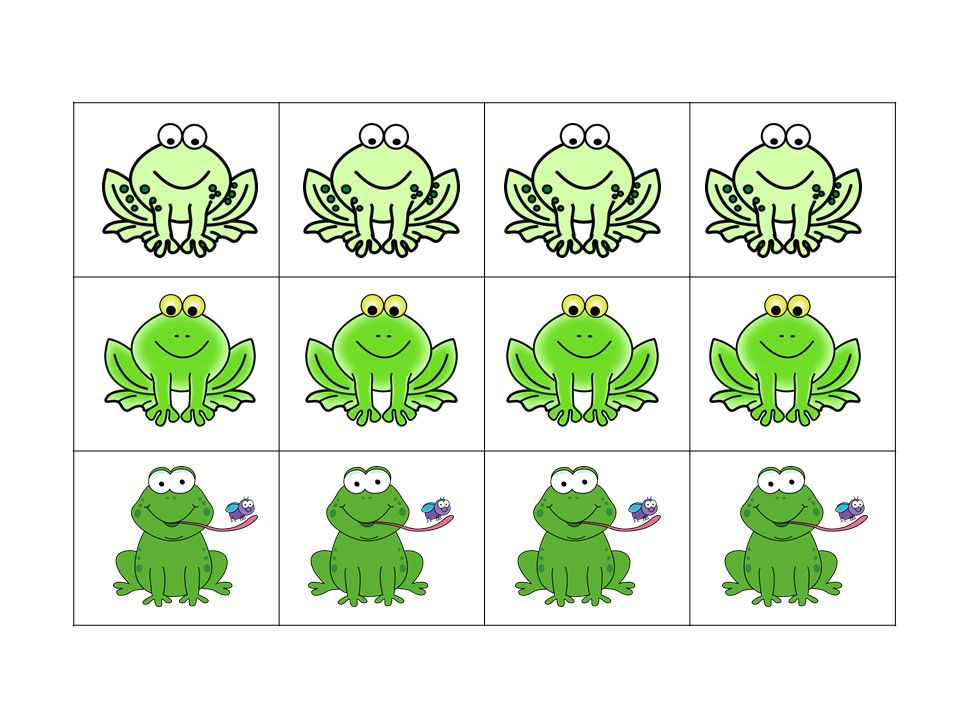top of page
Search
• Becky Carlisle Cothern

# How to Save Money on Math Mats and Manipulatives (FREEBIE included)

We know that using hands-on manipulatives in mathematics is crucial. But if you start looking around at manufactured math mats with manipulatives you can spend anywhere from \$20-\$40 when shopping on an educational site such as Lakeshore. How can a teacher afford to provide students with a variety math manipulatives to use throughout the year with prices this high? The answer is simple use printable math mats and manipulatives to practice counting, number representation, addition, subtraction, and other math problems.

With printable math mats changing math centers to match your thematic units is simple, easy, and cheap. Simply replace the math mats and manipulatives and use them to practice any math skill you are working on during the year. For example, use the ocean and the fish for a water theme, barn with chickens when talking about the farm, and cars on the road for transportation. The printable mats and manipulatives are fully colored and created with kid-friendly themes to engage students in every math lesson.So what makes math mats so crucial to have in every classroom? Mat mats provide hands-on interactions with math concepts. Students are able to visually see the concept as they create and manipulate the picture. Also math mats are versatile. Students can do so many things from sorting and patterning to counting, number representation, addition, subtraction, math problems, and much more.

Need some ideas to get you started using math mats here's some suggestions:

*Roll and count: Roll a die. Count the same number of manipulatives on the workmat as shown on the die.

*Numeral Match: Using a deck of number cards place a number card face up on the workmat. Count the manipulatives on the workmat to match the quantity with the numeral.

*Adding: Using a deck of number cards, draw two cards (place both cards on the workmat), count the quantity of manipulatives, and then add the two quantities together.

*Subtracting: Use two decks of cards. The first deck should be numbers 5-10. The second deck should be the numbers 0-5. Place a card from the first deck face up on the workmat. Count manipulatives to match the quantity. Draw a card from the second deck, place face up off the mat. Take manipulatives off the workmat to match the smaller number. How many are left?

*Word Problems: Make up a word problem using the workmat and manipulatives. Have students act out the word problem using the workmat and manipulatives. Solve the word problem.

Want to try printable math mats for FREE. Just click below to print a frog math mat you can use in your classroom next year. Enjoy!!!